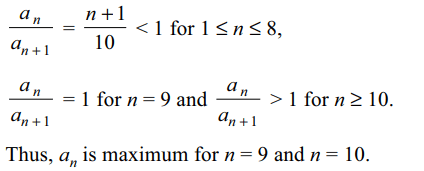## Permutations and Combinations Questions and Answers Part-11

1. If n is odd and $^{n}C_{0}<^{n}C_{1}<^{n}C_{2}< ...<^{n}C_{r}$     , then maximum possible value of r is
a) $\frac{1}{2}\left(n+1\right)$
b) $\frac{1}{2}\left(n-1\right)$
c) n
d) $\frac{1}{3}\left(n+1\right)$

Explanation:2. The number of arrangements of the letters of the word BANANA in which two N’s do not appear adjacently is
a) 40
b) 60
c) 80
d) 100

Explanation:3. The number of positive integral solutions of $x_{1}x_{2}x_{3}x_{4}x_{5}=840$     is
a) 3125
b) 6125
c) 6250
d) 7250

Explanation:4. The number of ways we can put 5 different balls in 5 different boxes such that at most three boxes are empty, is equal to
a) $5^{5}+5$
b) $5^{5}-10$
c) $5^{5}-5$
d) $5^{5}-4^{5}$

Explanation: Total number of ways - number of ways in which exactly four boxes remain empty

5. The number of ordered pairs of integers (x, y) satisfying the equation $x^{2}+6x+y^{2}=4$     is
a) 2
b) 4
c) 6
d) 8

Explanation:6. The number of the divisors of 20! is
a) 4140
b) 41040
c) 4204
d) 81650

Explanation:7. The letters of the word COCHIN are permuted and all the permutations are arranged in an alphabetical order as in an English disctionary. The number of words that appear before the word COCHIN is
a) 360
b) 192
c) 96
d) 48

Explanation: All the words beginning with CC, CH, CI and CN occur before COCHIN.

8. The number of five digit number that can be formed by using digits 1, 2, 3 only, such that exactly three digit of the formed number are same, is
a) 30
b) 60
c) 90
d) none of these

Explanation:9. The number of zeros at the end of 100! is
a) 20
b) 22
c) 24
d) 2610. Let $a_{n}=\frac{10^{n}}{n!}$    for n ≥ 1. Then $a_{n}$ take the greatest value when n is equal to